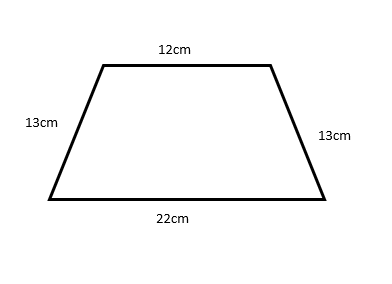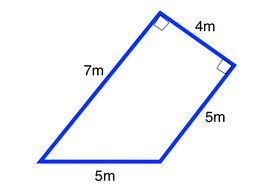Area of Trapezoid homework Help at TutorEye

# Best Homework Help For Area of Trapezoid

## Top Questions

t schedule 3 people for the day my % scheduled is 78.57% My question is how did the computer come up with the 78.57%?
View More

I would love to get a written solution. I did the other proof tree that you can see in the attaached files. So its supposed to look like that Thank You.
View More

xtbook. i really need in in half an hour
View More

## Area of Trapezoid:

A quadrilateral having one set of parallel sides and other sets of non-parallel sides is known as a trapezoid.

1/2 . (a+b) . h

Where , a and b are the length of parallel sides, and h is the height of the trapezoid.

## Area of Trapezoid Sample Questions:

Question 1: Calculate the area of a trapezoid whose height is 15cm and length of parallel sides are 8cm and 18cm?

(a) 185 sq.cm

(b) 190 sq.cm

(c) 195 sq.cm

(d) 180 sq.cm

Explanation: Use the area of trapezoid formula.

Question 2: If the lengths of the two parallel sides of the trapezoid are 17m and 25m and its area is 378sq.m. Find the height of the trapezoid?

(a) 18m

(b) 21m

(c) 20m

(d) 25m

Explanation: Use the area of trapezoid formula.

Question 3: A trapezoid has a base of length of 6cm, a length of another base be s, and  height be s.The sides of a square are of length s.What is the value of s such that the area of the trapezoid and the square are the same?

(a) 4

(b) 4√2

(c) 6

(d) 1

Explanation: Use the area of trapezoid formula.

Question 4: Find the area of trapezoid(a) 200 sq.cm

(b) 210 sq.cm

(c) 204 sq.cm

(d) 205 sq.cm

Explanation: Use the area of trapezoid formula.

Question 5: Find the area of given trapezoid?(a) 25 sq.m

(b) 26 sq.m

(c) 28 sq.m

(d) 24 sq.m

Explanation: Use the area of trapezoid formula.

Question 6: Rohit is building a stage, which is shaped like a trapezoid. The bases are 8m and 4 m, and the height is 20 m. How many square meters large is the stage?

(a) 120 sq.m

(b) 110 sq.m

(c) 140 sq.m

(d) 130 sq.m

Explanation: Use the area of trapezoid formula.

Question 7: The area of a trapezoid is 225cm². Its parallel sides are in the ratio 1 : 2 and the perpendicular distance between them is 15 cm. Find the length of each of the parallel sides of the trapezoid?

(a) 10 cm & 20 cm

(b) 15 cm & 30 cm

(c) 20 cm & 40 cm

(d) 25 cm & 50 cm

Explanation: Use the area of trapezoid formula.

Question 8: The area of a trapezoid is 220 cm² and its height is 20 cm. If one of the parallel sides is longer than the other by 8 cm, find the two parallel sides?

(a) 8 cm & 16 cm

(b) 7 cm & 15 cm

(c) 6 cm & 14 cm

(d) 5 cm & 13 cm

Explanation: Use the area of trapezoid formula.

Question 9: Find the height of a trapezoid whose area is 640 cm2 and its parallel sides are 13 cm and 27 cm?

(a) 34cm

(b) 32cm

(c) 36cm

(d) 38cm

Explanation: Use the area of trapezoid formula.

Question 10: A forested region in the shape of a trapezoid with bases of 128 metres and 92 metres and a height of 40 metres.The walkway is 4 metres wide and runs perpendicular to the two bases.Calculate the size of the forested area once the pathway has been added?

(a) 4200 m^2

(b) 4240 m^2

(c) 4220 m^2

(d) 4400 m^2